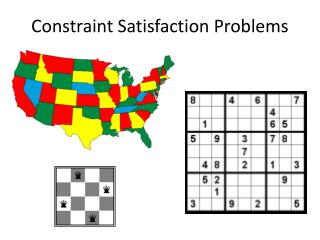# Constraint Satisfaction Problems - PowerPoint PPT PresentationDownload PresentationConstraint Satisfaction Problems

Constraint Satisfaction ProblemsDownload Presentation## Constraint Satisfaction Problems

- - - - - - - - - - - - - - - - - - - - - - - - - - - E N D - - - - - - - - - - - - - - - - - - - - - - - - - - -
##### Presentation Transcript

1. Constraint Satisfaction Problems

2. Constraint satisfaction problems (CSPs) • Standard search problem: • Stateis a “black box” – any data structure that supports successor function, heuristic function, and goal test • Constraint satisfaction problem: • Stateis defined by variablesXi with values from domainDi • Goal testis a set of constraints specifying allowable combinations of values for subsets of variables • A simple example of a formal representation language • Allows useful general-purpose algorithms with more power than standard search algorithms

3. Example: Map Coloring • Variables:WA, NT, Q, NSW, V, SA, T • Domains: {red, green, blue} • Constraints: adjacent regions must have different colorse.g., WA ≠ NT, or (WA, NT) in {(red, green), (red, blue), (green, red), (green, blue), (blue, red), (blue, green)}

4. Example: Map Coloring • Solutions are complete and consistent assignments, e.g., WA = red, NT = green, Q = red, NSW = green, V = red, SA = blue, T = green

5. Example: N-Queens • Variables:Xij • Domains: {0, 1} • Constraints: i,j Xij = N (Xij,Xik)  {(0, 0), (0, 1), (1, 0)} (Xij,Xkj)  {(0, 0), (0, 1), (1, 0)} (Xij,Xi+k, j+k)  {(0, 0), (0, 1), (1, 0)} (Xij,Xi+k, j–k)  {(0, 0), (0, 1), (1, 0)} Xij

6. N-Queens: Alternative formulation • Variables:Qi • Domains: {1, … , N} • Constraints: i, j non-threatening (Qi,Qj) Q1 Q2 Q3 Q4

7. Example: Cryptarithmetic • Variables: T, W, O, F, U, R X1, X2 • Domains: {0, 1, 2, …, 9} • Constraints: Alldiff(T, W, O, F, U, R) O + O = R + 10 * X1 W + W + X1 = U + 10 * X2 T + T + X2 = O + 10 * F T ≠ 0, F ≠ 0 X2 X1

8. Example: Sudoku • Variables:Xij • Domains: {1, 2, …, 9} • Constraints: Alldiff(Xij in the same unit) Xij

9. Real-world CSPs • Assignment problems • e.g., who teaches what class • Timetable problems • e.g., which class is offered when and where? • Transportation scheduling • Factory scheduling • More examples of CSPs: http://www.csplib.org/

10. Standard search formulation (incremental) • States: • Values assigned so far • Initial state: • The empty assignment { } • Successor function: • Choose any unassigned variable and assign to it a value that does not violate any constraints • Fail if no legal assignments • Goal test: • The current assignment is complete and satisfies all constraints

11. Standard search formulation (incremental) • What is the depth of any solution? • n (with nvariables assigned) • This is the good news (why?) • Given that there are m possible values for any variable, how many paths are there in the search tree? • n! · mn • This is the bad news • How can we reduce the branching factor?

12. Backtracking search • In CSP’s, variable assignments are commutative • For example, [WA = red then NT = green] is the same as [NT = green then WA = red] • We only need to consider assignments to a single variable at each level (i.e., we fix the order of assignments) • Then there are only mnleaves • Depth-first search for CSPs with single-variable assignments is called backtracking search

13. Example

14. Example

15. Example

16. Example

17. Backtracking search algorithm • Improving backtracking efficiency: • Which variable should be assigned next? • In what order should its values be tried? • Can we detect inevitable failure early?